# HBSE 9th Class Science Important Questions Chapter 9 Force and Laws of Motion

Haryana State Board HBSE 9th Class Science Important Questions Chapter 9 Force and Laws of Motion Important Questions and Answers.

## Haryana Board 9th Class Science Important Questions Chapter 9 Force and Laws of Motion

Question 1.
Define force.
Force is that external factor that changes or tends to change the position of rest or speed and direction of moving object.

Question 2.
What is balanced force?
When two or more forces acting on a body have zero resultant, then such forces are called balanced force.

Question 3.
What is unbalanced force ?
When the resultant of two or more forces is not zero, such forces are called unbalanced force.

Question 4.
Which force works when a rubber ball is pressed between palms ?
It is an example of a balanced force.

Question 5.
Which scientist has established the laws to control the motion of objects firstly?
Sir Isaac Newton.

Question 6.
What is the angle of inclination?
The angle made by an inclined plane with horizontal is called the angle of inclination.

Question 7.
Define frictional force.
It is the opposing force that comes between the two surfaces in contact when a body moves or tends to move on another body.

Question 8.
Write the effect of frictional force.
Frictional force reduces the motion of body.Question 9.
Why the bicycle stops when we stop pedaling?
The frictional force between the tyre of the bicycle and road reduces its motion and stops the bicycle.

Question 10.
Why powder is spread on the carom board in carom game?
The powder is spread on the carom board to reduce the frictional force between carom board and coin.

Question 11.
What is the law of conservation of momentum?
According to law of conservation of momentum, in absence of any external force, the total momentum of a system remains conserved.

Question 12.
Write Newton’s second law of motion?
The rate of change of momentum of an object is proportional to the applied unbalanced force in the direction of force. ‘

Question 13.
What is meant by momentum?
The momentum of an object is the product of mass and velocity. It is denoted by p.
or p = mv

Question 14.
What is SI unit of momentum?
Kilogram metre per second (kg ms-1).

Question 15.
Momentum is a scalar quantity or vector.
Momentum is a vector quantity, because it has both magnitude and direction.

Question 16.
In which direction, the momentum works ?
Momentum works in the direction of velocity.

Question 17.
What is the relation among force, mass and acceleration ?
Force = Mass x Acceleration (F = ma)Question 18.
What is the SI unit of force ?
The SI unit of force is Newton.

Question 19.
Define one Newton force.
One Newton force is that force which produces an acceleration of 1 ms-2 in a body of mass 1 kg.

Question 20.
What is negligible action ?
When a body affects the other by applying force, then we say that there is negligible action between first and second body.

Question 21.
How many types of inertia is ?
There is two types of inertia:
(i) Inertia of rest
(ii) Inertia of motion.

Question 22.
Write the concept of force on the basis of momentum.
F = $$\frac{p_2-p_l}{t_2-t_l}$$

Question 23.
How much force will be required to produce an acceleration of 2mS“2 in 6 kg object ?
Force (F) = Mass (m) x Acceleration (a) = 6 x 2 = 12 N

Question 24.
A person pushes a box of 40 kg with a force of 80 N. What will be the acceleration of box ?
Acceleration (a) =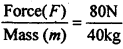= 2 ms-2

Question 25.
Write Newton’s third law of motion.
According to Newton’s third law of motion, “The action and reaction are always equal and in opposite direction.”

Question 26.
What is inertia ?
That property of objects which opposes the change in its motion or state of rest is called inertia.

Question 27.
Is the existence of a single force is possible ?
No, the existence of a single force is not possible. The force is always in pairs.

Question 28.
Which force offers us the motion in a desired direction ?
The force which offers us the motion in a desired direction is the reaction of the earth opposite to the force applied by our body.Question 29.
Are the action and reaction imposed on an object ?
No, action and reaction (force) are imposed on two different objects.

Question 30.
Write the name of two forces which arise without the contact of two objects.
(i) magnetic force
(ii) force of gravity.

Question 31.
How the fruit from tree falls towards the earth ?
Due to force of gravity.

Question 32.
Why is it difficult to climb on a smooth pole ?
Due to less reaction.

Question 33.
Why oil is used in machines ?
To reduce the friction.

Question 34.
Why is it difficult to walk on sand ?
Due to more friction between feet and sand.

Question 35.
Compare the frictional force between the surfaces of solids and liquids.
The frictional force between the surfaces of liquids is much less than the frictional force between the surfaces of solids.Question 36.
A small piece of stone and a truck are moving with same motion. On which, the larger force will be imposed to stop both of them ?
The larger force will be required to stop the truck, because it will have large momentum.

Question 37.
By which force, we can walk on road without slipping.
Due to frictional force, we can walk on road without slipping.

Question 38.
Why it becomes difficult to walk on a green mossy road in rainy season ?
The frictional force becomes less on mossy road so it becomes difficult to walk on such a road.

Question 39.
Which Newton’s law of motion helps us in the measurement of force ?
Newton’s second law of motion helps us in the measurement of force.

Question 40.
According to which Newton’s Law of motion, jet aeroplanes fly ?
They fly according to Newton’s third law of motion.

Question 41.
Why the player runs before jump ?
To increase the momentum so that the jump can be high.

Question 42.
Force is a scalar quantity or Vector.
Force is a vector quantity.

Question 43.
What is the total momentum of rifle and bullet after firing from rifle ?
Zero.

Question 44.
How much acceleration will be produced if 1 newton (N) force is applied on an object of 1 kg mass ?
1 ms-2Question 1.
What is meant by force ? What can be the effect on object by applying force on that ?
Force is that external factor which changes or tends to change the position of rest or speed and direction of moving object.
Effects:
Following are the effects on body, when force is applied :
(i) Speed of object can be changed due to which the object moves fast or slow.
(ii) The direction of motion of object can be changed and can move body in rest.
(iii) Shape of the object can be changed.

Question 2.
Give five examples of force in daily life.
Examples of force in daily life are as follows :
(i) When a force is applied on a rubber ball, it elongated. It is due to force.
(ii)When a spring is stretched, its length increases due to muscular force.
(iii) The steam gives its energy to steam engine due to that the steam engine moves forward.
(iv) A rolling ball can be stopped by muscular force.
(v) Petrol produces mechanical force by its energy, due to that bus, car etc. moves.

Question 3.
What is inclinded plane ? What are its uses ?
A smooth plane which makes an angle with horizontal plane is called inclinded plane, e.g. knife, axe, screw, hilly roads, stairs etc. A man lifting a heavy weight with the help of inclinded plane made of wooden piece is shown in below fi.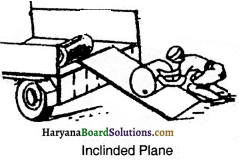Uses of inclinded plane:
The uses of inclinded plane are as follows:
(i) A heavy weight can be lifted by small force by using inclinded plane.
(ii) Heavy weight is loaded or unloaded in trucks by using it.
(iii) Screw also works on the principle of inclinded plane.

Question 4.
Distinguish between balanced and unbalanced forces.
The difference between balanced and unbalanced forces are as follows :
Balanced Force Unbalanced Force
1. When balanced forces act together on a body, their resultant is zero.
2. The balanced force can’t move a body which is in rest position.
3. This force cannot change the speed and direction of moving body.
4. This force always changes the size of body.

1. When unbalanced forces act together on a body, their resultant is not zero.
2. The unbalanced force can move a body which is in rest position.
3. This force can change the speed and direction of a moving body.
4. This force does not always change the size of body.

Question 5.
What is meant by inertia ? Clarify by example.
The property of bodies which opposes the change in motion of body or state of rest is called inertia.

Experiment:
Set a coin on a stiff playing card covering an empty glass tumbler. Give the card a sharp horizontal flick with a finger. You will see the card moves ahead but the coin will fall in glass. When the card is shoot fast, then due to property of inertia, the coin tries to remain in rest position. In this way, when the card moves, the coin falls in glass.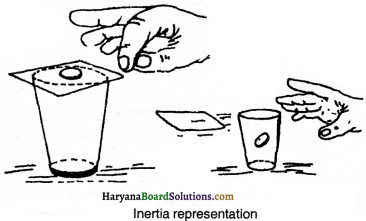Question 6.
What are the types of inertia ? Explain with example.
Inertia is of two types:
1. Intertia of rest: The property of body by which the body resists its rest position until an external force is not applied on it.
Example: A book on table does not move due to inertia of rest.2. Inertia of motion: The property of body by which it resists its state of motion, until an external force is not applied on it.
Example: A passenger sitting in a bus or cart falls forward when the moving bus or cart stops suddenly. In such situation, the lower portion of body of passenger comes in rest as the bus or cart stops, but the upper portion tries to remain in motion, due to this passenger falls forward. –

Question 7.
What happens when a wet cloth is jerked ? Clarify your explanation.
When we jerk the wet cloth, the cloth displaced from its position to inertia and they detached from cloth and escape in environment. Thus, the cloth dries fast.

Question 8.
In javelin throw, if a player crosses the fixed line, he fouls, but the playes usually fail to stop on this line, why ?
When in javelin throw competition, a player runs and reaches the fixed line, his whole body comes in motion. When he tries to stop his feet on fixed line, the upper portion remains in motion due to inertia of motion and the player crosses the fixed line and fouls.

Question 9.
Why the rider falls backward when the horse starts running suddenly?
The rider falls backward when the horse starts running suddenly because due to suddenly running the horse, it comes in motion but the rider tries to remain in rest due to inertia of rest and falls backward.

Question 10.
Why the rider falls forward when a running horse stops suddenly?
The rider falls forward when a running horse stops suddenly because the horse and rider are in motion when it is running and when horse
stops suddenly it comes in rest but the rider tries to remain in motion due to inertia of motion and falls forward.

Question 11.
The movement of vehicles is due to the frictional force between road and tyres. If their tyres are smooth then the friction will be less betw een road and tyre, so that it will be difficult to control the vehicle. In order to control vehicles, the tyres are made rough and treaded so that the friction between tyre and road increases and grip of tyres with road will be firm.

Question 12.
Define and explain Newton’s first law of motion.
Newton’s first law of motion:
An object continuous to be a state of rest or of uniform motion along a straight line unless acted upon by an unbalanced force. This law is also known as “Galileo’s Law of Inertia.” e.g. a ball rolling on floor can move far due to less friction. If the friction reduces to zero by any mean, the ball will move with initial velocity for a certain time.Question 13.
Prove that Newton’s first law of motion can be called the law of inertia.
According to Newton’s first law of motion, an object continuous to be a state of rest or of uniform motion along a straight line unless acted upon by an unbalanced force. In other word, all objects oppose the change in their state of motion. This tendency of the objects to oppose the change in their state of motion is called inertia. Thus, Newton’s first law of motion is also called the law of inertia.

Question 14.
Prove that the mass of a body is the measure of its inertia.
The quantity of matter in a body is its mass. In this way, the mass is the measure of inertia of body. A body having large mass has large inertia.

Explanation:
If we kick a football it moves so far. but if we kick a stone, it will not move and our foot gets wound, because in comparison of football, stone has larger capacity to oppose the change in motion due to its larger mass. The mass of a body is the measure of its inertia.Thus, the inertia of a stone is more.

Question 15.
Define and explain Newton’s second law of motion with the help of this law, obtain equation of force.
Newton’s second law of motion : The force applied on a body is proportional to the product of its mass and acceleration. If we kick a ball, it will move quickly because its acceleration becomes more. In this way, a cricketer stops a moving ball slowly, so that the change in motion of ball takes much time. He moves his hands in the direction of ball and the acceleration of ball becomes less in comparison of initial and less force is required to stop the ball. Let us consider a body has mass m and a force F produces an acceleration a in it. According to Newton’s second law of motion,
F ∝ m x a
or F = K ma ………..(i)
here, K , is a constant, whose value depends upon the units of mass, force and acceleration.
Let m = 1 kg, a = 1 m s-2 and f = 1 N
then, putting the value of F, m and a in eQuestion (i)
1 = K x 1 x 1
or K = 1
F = 1 x ma
Force = Mass x Acceleration

Question 16.
Define and explain Newton’s third law of motion.
Newton’s third law of motion:
To every action, there is an equal and opposite reaction and they act on two different bodies. Action and reaction are equal and in opposite direction.

For example:
(i) A ball presses the earth with a force, the earth pushes the ball upwards with same force.
(ii) When a person swims, he pushes the water backward, he moves forward, by this reaction.Question 17.
Explain why does cricket player pulls his hands backward while catching a fast moving cricket ball ?
The momentum of moving ball is more. When player catches the ball, he pulls his hands backward so that momentum of ball takes some time to become zero. In this way, momentum of ball, rate of change and the force applied on hand will become less and there is no hurt on hands.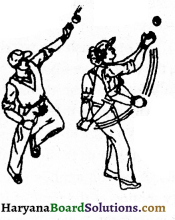Question 18.
Why larger force is required to stop a ship than car moving on road ?
The frictional force between the tyres of car and road is more. Therefore the car stops quickly by applying lesser force. The frictional force between the surfaces of liquids is much less than the frictional force between the surfaces of solids. Due to this reason, the ship experiences less friction in water. Thus, larger force is required to stop a ship than car.

Question 19.
When there is negligible reaction between two bodies how the direction changes when two moving objects strike? Clarify with figure.
When there is negligible reaction between two bodies, one body affects the other by force. The collision between two marbles is an example of negligible reaction. In figure two marbles are shown moving towards each-other. The direction of their motion is shown by arrow. It is clear from figure that the direction of marble changes after collision. Two forces act in this action. One is that which has accelerated one marble and second is that which has accelerated the other marble.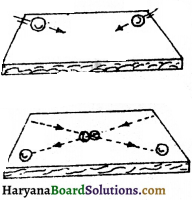Question 20.
What is meant by action and reaction ? What is the relation between them ? In which law of motion, these are described ?
If a body applies some force on other, then it is the active force on other body. In response, the force which second body applied on first is the reactive force on first body. The relation between action and reaction : The force of action and reaction are equal and in opposite directions. An important fact is that the force of action and reaction always act on different bodies. These are described in third law of motion.

Question 21.
Give some examples of Newton’s third law of motion in daily life.
Following are the examples of Newton’s third law of motion :
(i) The bullet moves forward when trigger is pressed and due to reaction force, the gun recoils backward.
(ii) The person pushes the water backward while swimming and moves forward due to tne reaction or
water.
(iii) When a person jumps from boat at river-shore, the boat displaced far from shore due to reaction of water on that.
(iv) We press the earth backward while walking and
due to force of reaction the earth pushes our feet forward and we move. .
(v) The ores push the water backward and due to reaction, the boat move forward.
(vi) When a player jumps up, he pushes the surface of earth downward, so that he gets reaction.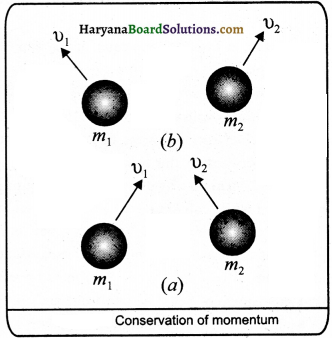Question 22.
Why the boat moves in opposite direction when a person jumps to shore from the boat ? Explain.
A person applies force in upward to get the necessary motion at the time of jumping from boat. His reaction is on boat, which according to Newton’s third law of motion is in opposite direction. As a result of this, the boat move far on opposite direction.Question 23.
Explain in brief, the relation between Newton’s first and second law of motion.
The Newton’s first law of motion is the part of second law. It can be clarify on the basis of following fact:
According to second law of motion,
F = m a
if F = 0, then
m a = 0 m = 0,
a = 0
But mass, m cannot be zero, because it is a constant quantity. So, if acceleration a is zero, the change in velocity will also be zero. Therefore, there is no change in the position of body. If it is in rest, it will remain in rest, and if it is in motion, it will remain in motion unless external force is not applied. This is the statement of Newton’s first law of motion. Thus Netwon’s first law of motion is the part of second law.

Question 24.
What is the reason that a fast moving bullet makes a round hole in glass but on throwing the stone, the glass damages ?
The size of bullet is small and that of stone is big so that the glass does not move when bullet is striked, only that part move where the bullet is striked and makes a hole, but there is no hole when stone is striked because whole glass moves and damaged.

Numerical Questions

Question 1.
Speed-time graph of a moving coin on table is shown in fig. . The weight of coin is 10 g.
How much force the table exerts to stop the coin?
Solution:
Here,
Initial velocity (u) = 24 ms-1
Final velocity (v) = 0 ms-1
Time (r) = 8 sec
Mass (m) = 10g = $$\frac {10}{1000}$$ kg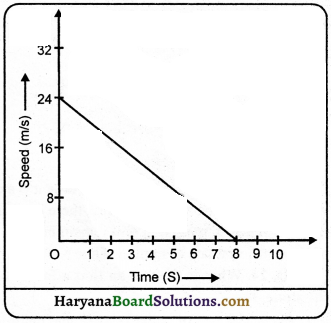We know that,
Acceleration (a) = $$\frac{v-u}{t}=\frac{0-24}{8}$$ = $$\frac {-24}{8}$$ = -3 ms-2
∴ Force(F) = m.a
$$\left(\frac{10}{1000} \times-3\right)$$ N
= – 0.03 N
(- ve sign shows that force decreases the motion.)

Question 2.
A cricket ball of mass 70g is moving with a velocity of 0.5 ms-1. A cricket player stops it in 0.5 s. Calculate the force applied by the player to stop
the ball.
Solution:
Mass of the ball (m) 70g =$$\frac {70}{1000}$$= 0.07 kg
Initial velocity of ball (u) = 0.5 ms-1
Final velocity of ball (v) = 0
The time taken to stop the ball, (t) = 0.5s
We know that, v = u + at
a = $$\frac{v-u}{t}=\frac{0-0.5}{0.5}=\frac{-0.5}{0.5}$$ = -1 ms-2
Thus, the force applied by the player to stop the ball
(F) = ma
= 0.07 x -1N = -0.07 N
(-ve sign shows that force is working against momentum.)

Question 3.
How much acceleration will be produced in the object ¡fa force of 200 N is applied on an object of 5 kg mass?
Solution: Here,
Mass(m) = 5kg
Force (F) = 200N
Acceleration (a) = ?
We know that, F = ma
a = $$\frac{F}{m}=\frac{200}{5}$$ = 40 ms-2Question 4.
A constant force is working on an object of 5 kg mass for 2 seconds. It increases the velocity of object from 3ms1 to 7ms-1. Calculate the value of applied force. Now, what will be the final velocity if the force works for 5 seconds on object?
Solution:
Here,
Mass of object (m) = 5 kg
Initial velocity of object (u) = 3 ms-1
Final velocity of object (v) = 7 ms-1
Time (t) = 2s
Acceleration (a) = ?
We know that.
a = $$\frac{v-u}{t}=\frac{7-3}{2}$$ms-2 = $$\frac {4}{2}$$ = 2 ms-2
The force applied on object (F) = ma
= 5 x 2 = 10 N

In second stage,
Initial velocity of object (u) = 3 ms-1
Final velocity of object (v) = ?
Time (t) = 5s
Acceleration (a) = 2 ms-2
We know that,
v = u + a = 3 + 2 x 5 = 13 ms-1

Question 5.
How much force is required to stop a car of mass 1000 kg and a loaded truck of mass 10,000 kg moving both with a velocity of 5ms in 2 seconds?
Solution:
Here.
Initial velocity of both (u) = 5ms
Final velocity of both (v) = O
time (t) = 2 s
Acceleration (a) = ?
We know that, v = u + at
a = $$\frac{v-u}{t}=\frac{0-5}{2}=\frac{-5}{2}$$ = ms-1

Mass of the car (m1) = 1000 kg
Mass of the truck (m2) = 10,000 kg
The necessary force to stop the car (F1) = m1 a
= 1000 x $$\frac {-5}{2}$$ N
= – 2500 N
Thus, necessary force to stop the car = – 2500 N
(-ve sign shows that force is working against momentum.)
In same way, the necessary force to stop the truck (F2) = m2 a
= 10000 x $$\frac {-5}{2}$$N
= – 25000 N
Therefore, the necessary force required to stop the truck is more than that of car.Question 6.
Which will require more force – to accelerate a body of mass 2 kg with a rate of 5 ms-2 or to accelerate a both of mass 4kg with a rate of 2 m-2?
Solution:
Here,
m1 = 2kg
a1 = 5 ms-2
Force (F1) = m1a1 = 2 x 5 = 10 N
m2 = 4 kg
a2 = 2 ms-2
Force(F2) = m2a2 = 4 x 2 = 8N
In this way F1> F2
∴ Large force will required to accelerate the object of 2 kg mass with a velocity of 5 ms-2

Question 7.
A bullet of mass 20 g is horizontally fired with a velocity of 150 ms from a pistol of mass 2 kg. What is the recoil velocity of the pistol?
Solution:
Mass of bullet, m1 = 20 g ( = 0.02 kg)
Mass of pistol. m2 = 2 kg
The initial velocity of bullet (u1) and pistol (u2) is zero, respeciively.
That is u1 = u2 = 0
Final velocity of bullet, v1 = + 150 ms-1
The direction of bullet is taken + ve according to left to right system. Suppose, recoil velocity of pistol is v.
Total momentum of bullet and pistol before firing = (2 + 0.02) kg x o ms-1
= 0 kg ms-1
Total momentum after firing = 0.02 kg x (+ 150 ms-1) + 2 kg x v ms-1
= (3 + 2v)kg ms-1
According to the law of conservation of momentum,
Total momentum after firing = Total momentum before firing
3 + 2v = 0
2v = -3
v = $$\frac {-3}{2}$$
v = -1.5 ms-1
(-ve sign shows that the pistol recoils opposite to the direction of bullet i.e. right lo left.)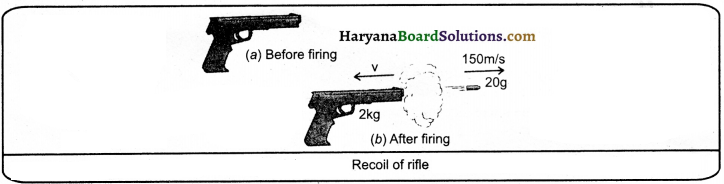Question 8.
A coin is moving with a velocity of 24 cm s rolls with an acceleration of 3 cm2 and stops in 8 s. Find the distance covered by the coin.
Solution:
Here,
Initial velocity (u) = 24 cm s
Final velocity (v) = 0
Acceleration (a) = – 3 cm s2
Time (t) = 8s
Distance (s) = ?
We know that, Distance (s) = ut + $$\frac {1}{2}$$ at
= 24 x 8 + $$\frac {1}{2}$$ x (-3) x (8)2
= 192 – 96
= 96 cmQuestion 9.
In which the more force will require-to produce an acceleration of 5 ms in mass 10 g or to produce an acceleration of 2 ms2 in mass 20 g?
Solution:
In first stage, Mass (m) = 10 g = $$\frac {10}{1000}$$ kg
Acceleration (a) = 5 ms-2
The necessary force (F) = m x a
= $$\frac {10}{1000}$$ x 5
= 0.05 N
In second stage, Mass (m) = 20 g = $$\frac {20}{1000}$$ kg
Acceleration (a) = 2 ms-2
The necessary force (F) = m x a = $$\frac {20}{1000}$$ x 2 = 0.04N
Thus, larger force will be required to produce an acceleration of 5 ms2 in an object of mass 10 g. Answer:

Question 10.
A bullet of mass 20 g moving with a velocity of 16 ms-1 enters ¡n a sand bag and comes in rest after 0.05 s. Find,
(a) depth of penetration ¡n sand
(b) the average resistive force applied by sand.
Solution:
Here,
(a) Initial velocity (u) = 16 ms-1
Final velocity (v) = 0
Time (t) = 0.05 s
Distance (s) = ?
Average resistive force (F) = ?

We know that,
Acceleration (a) = $$\frac{v-u}{t}=\frac{0-16}{0.05}$$ = 0.05
= $$\frac{v-u}{t}=\frac{0-16}{0.05}$$
= $$\frac{-16 \times 100}{5}$$ = – 320 ms-2
s = ut + $$\frac {1}{2}$$ at2
= 16 x 0.05 + $$\frac {1}{2}$$ x (-320) x (0.05)2
or s = 0.8 – 0.4 = 0.4m
(b) Mass (m) = 20 g = $$\frac {20}{1000}$$ kg
Average resistive force (F) = m x a = $$\frac {20}{1000}$$ x -320 = -6.4 N

Question 11.
How much will be the change in momentum of an object of mass 5kg, If Its speed:
(i) reduces from 20 ms-1 to 0.20 ms-1
(ii) Increases from 30 ms1 to 40 ms-1.
Solution:
Here,
Mass of the body (m) = 5kg
Initial velocity (u) = 20 ms1
Final velocity (v) = 0.20 ms1
Initial momentum (p1) = m x u = 5 x 20 = 100 kg ms-1
Final momentum (P2) = m x v = 5 x 0.20 = 1.00 kg ms-1
Thus, decrease in momentum = p1 – p2 = 100 – 1 = 99 kg ms-1
Initial velocity (u) = 30 ms1
Final velocity (v) = 40 ms1
initial momentum (p1) = m x v = 5 x 30 = 150 kg ms-1
Final momentum (p2) = m x v = 5 x 40 = 200 kg ms-1
Thus, increase in momentum = 200 – 150 = 50 kg ms-1

Question 12.
A driver accelerates a car In first stage with 1.8 ms-2 and in second stage with 1.2 ms-2 acceleration. Find the ratio of forces applied by engine in both stages.
Solution:
Let,
The mass of car = m kg
Acceleration in first stage = (a1) = 1.8 ms-2
∴Force in first stage (F1) = m x a1 = m x 1.8m = 1.8 m N
Acceleration in second stage (a2) = 1.2 ms-2
∴Force in first stage (F2) = m x a2 = m x 1.2 = 1.2m N
$$\frac{F_1}{F_2}=\frac{1.8 \mathrm{~m}}{1.2 \mathrm{~m}}=\frac{18}{12}=\frac{3}{2}$$
F1:F2 = 3:2Question 13.
On applying a force for 1.2 s on an object, its speed increases from 1.8 ms-1 to 4.2 ms-1. The same force is applied for 2 s again. How much change in speed will be in 2s?
Solution:
Initial velocity in first stage (u) = 1.8 ms-1
Final velocity (v) = 4.2 ms-1
Time (t) = 1.2 s
According to formula, v = u + at
or u + at = v
1.8 + a x 1.2 = 4.2
or 1.2a = 4.2 – 1.8
or 1.2a = 2.4
or a = $$\frac {2.4}{1.2}$$ = 2 ms-1
∴ Acceleration = 2 ms-2
initial velocity in second stage (u) = 4.2 ms
Time (t) = 2s
Acceleration (a) = 2 ms2
Final velocity (v) = u + at = 4.2 + 2 x 2 = v = 4.2 + 4 = v = 8.2 ms-1
Thus, change in speed in 2s = 8.2 – 4.2 = 4 ms-1

Do Yourself

(1) How much force will require to produce an acceleration of 2ms2 in an object of mass 12 kg? What
will be the acceleration, if force is doubled?
24N, 4 ms-2

(2)Aperson pushes a box of mass 50kg by a force of 80 N. What will be the acceleration of box ? What will be the acceleration of box if its mass is reduced to half?
1.6 ms-2, 3.2 ms-2

(3) A motorcycle is moving with a velocity of 90 kmh1 and on applying brakes, stops in 5s. Calculate the force applied by brake on motorcycle, if its mass including driver is 200 kg.
1000 N

(4) A rifle of mass 3 kg fires a bullet of mass 0.03 kg. The bullet leaves the barrel of the rifle at a velocity of 100 ms. ¡f the bullet takes 0.003 second to move through the barrel, calculate the force experienced by the rifle due to its recoil.
1000 N(5) Calculate that force which offers a velocity of 30 ms in lOs to a car, if the mass of car is 1500 kg.
4500 N

(6) A car starts from rest and moves for 2 minutes with an uniform acceleration of 0.1 ms2. Calculate the distance covered by the car.
720 m

(7) Calculate that force which will produce an acceleration of 0.40 ms-2 in a toy car of mass 0.25 kg.
0.1N

(8) An object is dropped froma height of 20 metres. 1f g = 10 ms-2 then, calculate the velocity when it strikes to earth. Find the time taken to fall from that height.
20 ms-1, 2s

(9) The velocity-time graph of a ball of mass 20 g moving in straight line on a long table is giyen in figure. How much force will be table offer to ball to take it in rest?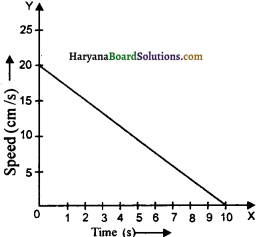0.0004 N

(10) A bullet of mass 10 g leaves the barrel with a velocity of 300 ms1 in 0.0035 s. How much force was exerted by the rifle on bullet?
857.14 NJ

(11) A car is moving with a speed of 108 kmh1 and takes 4s to stop after applying brakes. Calculate the force on car after applying brakes. Total mass of car including passengers, is 1000 kg.
750 N

(12) A force of SN produces an acceleration of 10 ms-2 in mass m1 and 20 ms-2 in mass ms-2. What will be the acceleration produced by this force, if both the masses are combined?
6.67 ms-2

(13)A resultant force of 15 N is applied on an object kept on a frictionless horizontal plane produces an acceleration of 5 ms-2. Find the mass of object.
3kg

(14) On applying a force of 5 N on mass in1, an acceleration of 8 ms2 is produced and by applying same force on mass ms-2, produces an acceleration of 24 ms-2 . How much acceleration will be produced by in combined mass by this force. 1f both masses becomes one by tieing?
6 ms-2(15) Two hockey players of opposite teams, while trying to hit a hockey ball in the ground collide and immediately get entangled. One has a mass of 60 kg and is moving with a velocity of 5 ms-2 while the other has a mass of 55 kg and is moving faster with a velocity of 6 ms towards the first player. In which direction and with what velocity will they move after they get entangled ? Assume the frictional force between the feet of the two players and the ground is negligible.
Right to left and with velocity of 0.26 ms

Essay Type Questions

Question 1.
How Galileo proved that the object will move with a ceaseless speed if a balanced force is not applied on that object?
On the basis of experiments written ahead, Galileo first of all told that the object will move with a ceaseless speed if a balanced force is not applied on that object. Galileo studied the motion of objects on a double inclined plane. He observed that velocity of a body increases when it rolls down the inclined plane and decreases when it climbs upon as shown in fig.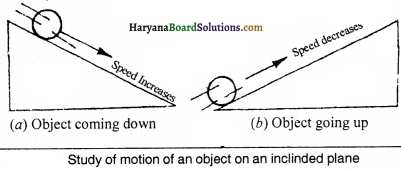Thus, he concluded that if the body moves on smooth plane then its speed neither increases nor decreases but its speed will remain ceaseless.
Now, he imagine that if a ball is allowed to go down by touching a hollow semi-spherical surface, it will reach the same height to which it was dropped. He concluded on the basis of motion of bob pendulum because the bob of pendulum attains that height from where it started moving. Semi-spherical surface works the same which thread does in pendulum.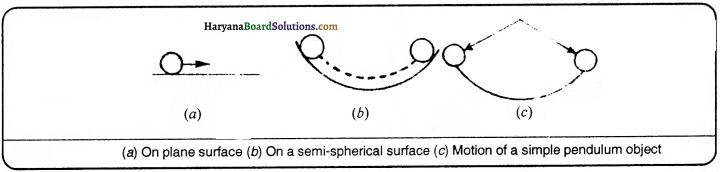After that he told that the shape of pot is taken as showm in fig., then the ball or object reaches the same height in this situation also. Yet, it has to cover large distance to do so.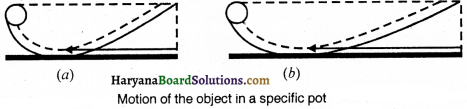Now, if the slope on the other side of pot is decreased, the ball covers the more distance to reach that height. In this way he concluded that if the slope is decreasing to one side of pot, the ball will cover the large distance. If there is no slope the surface becomes plane the ball will cover infinite distance and move with constant velocity.

Question 2.
What is the momentum of body ? Establish a relation between the momentum and force of a moving body.
Momentum: The momentum of body is the product of its mass (m) and velocity (v). It is denoted by ‘p’
Momentum = Mass x Velocity
or p = mv
We know that a body accelerates if some force is applied to it. According to Newton’s second law of motion, the acceleration produced in a body depends upon the force applied on that. If by applying a force, ‘F’ on a body of mass m, produces an acceleration of ‘a’ then according to second law of motion
F = ma
a = $$\frac {F}{m}$$ ………….(1)
Suppose, Initial velocity of body = v1ms-1
After time (t) the final velocity = v2 ms-1
Then a = $$\frac{v_2-v_1}{t}$$
From eqs. (i) and (ii) is
$$\frac{F}{m}=\frac{v_2-v_1}{t}$$
F = $$\frac{m\left(v_2-v_1\right)}{t}$$
F = $$\frac{m v_2-m v_1}{t}$$
But mv1 = p1 (Initial momentum)
and mv2 = P2 (Final momentum)
F = $$\frac{p_2-p_1}{t}$$
F = rate of chance of momentum.
Thus, the force applied on a body is equal to the change in momentum in unit time.Question 3.
What do you understand by conservation of momentum ? Prove the law of conservation of momentum in mathematical way.
Conservation Law of Momentum : According to this law, the total momentum of a group of objects remains constant until it is not act upon by external force.
Activity: To prove this law, suppose two objects A and B of mass m1 and m2 are moving with velocities u1 and u2 respectively. Let both react with each other till time t. After action, their velocities become v1 arid v2as
shown in fig.
if any external force does not act, then the rate of change in momentum of object A is m1 $$\frac{\left(v_1-u_1\right)}{t}$$ In the same way the rate of change in momentum of object B is m2 $$\frac{\left(v_2-u_2\right)}{t}$$ If a force F1 is exerted on A by B and F2 on B by A, then according to Newton’s second law of motion,

F1 = m1$$\frac{\left(v_1-u_1\right)}{t}$$ ………….(1)
and F2 = m2$$\frac{\left(v_2-u_2\right)}{t}$$ ………….(2)
According to Newton’s third law of motion, F1 and F2 act equally and ¡n opposite direction.
F1 = -F2 ………….(3)
From eqs. (1), (2) and (3), is
m1 = $$\frac{\left(v_1-u_1\right)}{t}$$ = m2
or m1(v1 – u1) = m2 (v2 – u2)
or m1v1 – m2u1 = m2v2 + m2u2
or m1v1 + m2v2 = m1u1 + m2u2
or m1 u1 + m2u2 = m1v1 + m2v2 ………(4)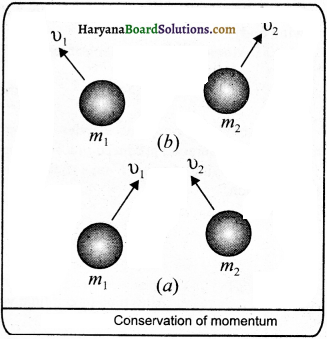Thus, total momentum before collision m1u1 + m2 u2 is equal to total momentum after collision m2v2 . This is the law of conservation of momentum.

Question 4.
What is meant by friction ? Prove that it is a boon as well as curse.
Friction: The opposing force that comes into play when a body moves on another surface or through a fluid (liquid or gas) is called friction.
Friction as a boon: The friction is a necessary evil because nothing is possible without it, that is:
(i) We cannot move if there is no friction between road and feet.
(ii) The vehicles cannot move if there is no friction between road and tyres.
(iii) The nail can be insisted in wall only due to friction.
(iv) Only due to friction, the match stick burns.
(v) It is possible to write on blackboard due to friction between chalk and blackboard.
(vi) Due to friction, we can write on copy.
(vii) The vehicles can be stopped only by friction.
(viii) The belts related to electric motors can move on wheels due to friction.Friction as a curse: Besides a necessary evil, friction is harmful to us as:
(i) Due to friction the parts of machine get bear and tear.
(ii) Due to friction, the efficiency of machines is not 100%.
(iii) More energy is spent due to friction.
(iv) Due to friction, our shoes tear.
(v) Due to friction, the tyres of vehicles reduces.

Question 5.
Write the methods of reducing friction. Explain any one out of them.
Methods of Reducing Friction : Friction can he reduced by following methods:
(i) The frictional force can be reduced by planing the depresions on the surface of body
(ii) Oil or lubricant educes the friction between two surfaces. Due to this the oil is put in the part machines and the friction can be reduced.
(iii) The mixture of water and soap can minimise the friction between two surfaces.
(iv) The friction can be minimize by using ball-bearings.
(v) When we sprinkle powder on a carom board, the powder tills up the depressions and the coin
(vi) In order to reduce friction, special shape is given to vehicles.
(vii) By polishing two surfaces and by putting coaltar on roads, the friction can be reduced.

To reduce the friction by using ball-bearIng : Ball-bearings are used in machines to reduce friction. Small balls are there in ball-bearings which are kept between the sliding surfaces of machines. You will have seen them in the wheels of cycle. These reduces the friction which save energy and work.

Practical Work ‘ .

Activity I.
Two activities to show the effect of force which proves that the force can change the size of body.

Procedure:
(i) Take a spring and pull it towards outside by holding its two ends. You, will see the size of spring increase, which shows the force applied on spring by us. It proves that the force can change the size of body.
(ii) Take a spherical rubber ball and press it between your palms. You will see that on pressing, the size of ball becomes some long. It proves that the force can change the size of body.

Activity 2.
Perform on activity to show that a body starts moving only when the force applied by us is more than frictional force.

Procedure:
Take a box and tell to children to push it. You will see that the box does not move if they apply less force, because the frictional force between the lower surface of box and rough surface of floor balances the force applied to push and due to this the box does not move. The children push the box with large force, but box does not move. This is because of that the friction force is still balances the pushing force. If the children push the box with much more force, then pushing force will become more than frictional force. Here, the unbalanced force is working and the box is moving. It proves that if the force applied by us is more than frictional force, the body moves.Activity 3.
Perform an activity to show the effect of action and reaction. .

Procedure:
(i) Take a big rubber balloon and inflate it fully.
(ii) Tie its neck using a thread. Also using adhesive tape, fix a straw on the surface of this balloon.
(iii) Pass a thread through the straw and hold one end of the thread in your hand or fix it on the wall.
(iv) Now remove the thread tied on the neck of balloon.
(v) Now the air escape from the mouth of the balloon and the straw and balloon will move in opposite direction which shows reaction.

Procedure 2:
(i) Take a test tube of good quality glass material and put a small amount of water in it.
(ii) Place a stop cork at the mouth of it. Now suspend the test tube horizontally by two strings.
(iii) Heat the test tube with a burner until water vaporises and the cork blows out.
(iv) We observe that the test tube recoils in the direction

Quick Review of the Chapter

1. When a force is applied on a body, its :
(A) motion can change
(B) size can change
(C) direction of motion can change
(D) all of the above
(D) all of the above

2. If the resultant of all forces acting on a body is zero, then all forces acting on body is called :
(A) balanced force
(B) unbalanced force
(C) frictional force
(D) none of these
(A) balanced force

3. Unit of force is:
(A) joule
(B) metre per second square
(C) Newton
(D) metre per second
(C) Newton

4. If the resultant of all forces acting on a particle is not zero, then forces are called:
(A) balanced force
(B) unbalanced force
(C) frictional force
(D) none of these
(B) unbalanced force

5 Which scientist has established the laws of controlling the motion of objects firstly ?
(A) Sir Isaac Newton
(B) C.V. Raman
(C) Rutherford
(D) Neils Bohr
(A) Sir Isaac Newton6. Newton’s laws of motion are:
(A) 2 ‘
(B) 3
(C) 4
(D) 5
(B) 3

7. Newton first law of motions is called:
(A) Law of friction
(B) Law of conservation of momentum
(C) Law of inertia
(D) Law of gravity
(C) Law of inertia

8. The rate of change of momentum of a body is proportional to the force applied and in the direction in which force is applied. This is Newton’s:
(A) first law
(B) second law
(C) third law
(D) fourth law
(B) second law

9. The product of mass and velocity of an object is called:
(A) momentum
(B) inertia
(C) friction
(D) force
(A) momentum10. SI unit of momentum is:
(A) kg-2
(B) kg ms-1
(C) kg m
(D) kg mh-1
(B) kg ms

11. The relation among F, m and a is:
(A) F = $$\frac {m}{a}$$
(B) Fa = m
(C) F = ma
(D) none of these
(C) F = ma12. The force required to produce an acceleration of 1 mc2 in a body of mass 1 kg is:
(A) 1 Newton
(B) 2 Newton
(C) 3 Newton
(D) 4 Newton
(A) I Newton

13. The force which acts without the contact of two objects is:
(A) magnetic force
(B) inertia
(C) friction
(D) all of the above
(A) magnetic force

14. According to which force, the apple faits on earth from tree?
(A) force of gravity
(B) inertia
(C) force of magnet
(D) frictional force
(A) force of gravity

15. It is difficult to walk on sand because the friction between feet and sand is:
(A) less
(B) more
(C) normal
(D) not there
(B) more16. A piece of stone and a truck is moving with same speed. In order to stop both, which one will require more force to stop?
(A) truck
(B) stone
(C) none on them
(D) both require equal.
(A) truck ‘

17. Which of the following force move the object?
(A) Gravitational force
(B) Unbalanced force
(C) Balanced force
(D) Frictional force
(B) Unbalanced force

18. Why the lubricant is used in machines?
(A) To increase friction
(B) To decrease friction
(C) To zero friction
(D) To clarity
(B) To decrease friction

19. The dust detached from carpet when beaten with stick because of:
(A) inertia of rest
(B) motion of carpet
(C) motion of stick
(D) force
(A) inertia of rest

20. If an external force ¡s working on a body, It accelerates in the dxrection of force. The acceleration produced is proportional to:
(A) applied force
(B) velocity
(C) mass
(D) inertia
(A) applied force

21. If the mass of two objects A and B is 6 kg and 34 kg respectively, then:
(A) the inertia of object A is more than B
(B) the inertia of B is more than A
(C) inertia of A and B are same
(D) inertia of A and B are zero
(B) the inertia of B is more than A

22. According to Newton’s third law of motion, the force related to action and reaction should apply on:
(A) one body
(B) same bodies
(C) always different bodies
(D) is not necessary to have equal result but their direction should be same
(C) always different bodies23. The explanation of falling forward when get down from bus can be done by:
(A) Newton ‘s first law of motion
(B) Newton ‘s second law of motion
(C) Newton’s third law of motion
(D) all of the above
(A) Newton’s first law of motion

24. Launching of rocket ¡s based on the principle of:
(A) Newton’s first law of motion
(B) Newton’s second law of motion
(C) Newton’s third law of motion
(D) all of the above
(C) Newton’s third law of motion

25. If the mass of an object m, velocity y, acceleration a, then the momentum (p) will be:
(A) p = mv
(B) p = $$\frac {m}{a}$$
(C) p = ma
(D) p = $$\frac {m}{a}$$
(A) p = mv

26. Which one of the following has mo inertia?
(A) a rubber ball
(B) a piece of stone
(C) a cycle
(D) a train
(D) a train27. The branch of a tree sheds its leaves when it is shedding fastly:
(A) due to mass
(B) due to inertia
(C) due to weight
(D) due to acceleration
(B) due to inertia

28. If an object of mass 30 kg has the momentum of 120 kg ms’, then its velocity will be:
(A) 4 ms-1
(B) 8 ms-1
(C) 2 ms-1
(D) 1 ms-1
(A) 4 ms-1

29. When a running bus stops suddenly, you falls forward and when starts running suddenly from rest you falls backward, It Is
(A) due to mass
(B) due to weight
(C) due to motion
(D) due to inertia
(D) due to inertia

30. The mass of a cart is 1500 kg. If the cart is to bring in rest with negative acceleration (retardation) of 1.7 ms-2, then the force between the cart and road will be
(A) 882.35 N
(B) 2550 N
(C) 1500N
(D) 2550 kgm
(B) 2550 N

31. A bullet of mass 10 g moving in a straight line with a velocity of 150 ms-1 strikes with a wooden piece and stops after 0.03 s. How far the bullet will penetrate the wood?
(A) 2.25 km
(B) 2.25 m
(C) 22.5m
(D) 15m